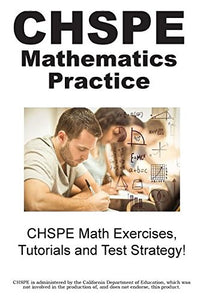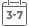# Chspe Mathematics Practice!: Chspe Math Exercises, Tutorials And Test Strategy!

• Publish Date: 2016-09-21
• Binding: Paperback
• Author: Complete Test Preparation Inc
Regular price \$39.98 Sale price \$35.91

Attention: For textbook, access codes and supplements are not guaranteed with used items.Over 200 CHSPE(R) math practice questions, prepared by a dedicated team of exam experts, with detailed answer key, Math shortcuts, tips and tricks, tutorials and multiple choice strategies!

CHSPE(R) Math Practice Questions and Tutorials for:

Numbers and Operation

• Scientific Notation
• Estimation
• Order of Operation

Patterns and Algebra

• Calculate the slope of a line
• Solve real world problems with ratio and proportion
• Analyze and calculate linear relationships
• Identify linear equations from a graph
• Solve problems involving patterns
• Identify and solve quadratic equations given values or graphs '
• Solve quadratic and linear equations
• Translate real world problems into quadratic equa-tions and solve

Data, Statistics and Probability

• Analyze and draw inferences from a set of data
• Make predictions based on data
• Calculate permutations and combinations
• Calculate dispersion and central tendency
• Calculate simple probability

Measurement and Geometry

• Calculate perimeter, circumference and volume
• Use scale on a map to calculate distances
• Solve problems using the Pythagorean theorem
• Determine geometric transformations
• Solve real world problems using the properties of geometric shapes

CHSPE(R) is a registered trademark of American Council on Education Corporation, who are not involved in the production of, and do not endorse this publication.

Practice Really Does Make Perfect!

The more questions you see, the more likely you are to pass the test. And with our practice questions, you'll have over 200 practice questions that cover every category.

Our CHSPE(R) Math practice test questions have been developed by our dedicated team of experts. All the material in the study guide, including every practice question, are designed to engage your critical thinking skills needed to pass the test!

Heard it all before?

Maybe you have heard this kind of thing before, and don't feel you need it. Maybe you are not sure if you are going to buy this book.

Remember though, it only a few percentage points divide the PASS from the FAIL students!

Even if our test tips increase your score by a few percentage points, isn't that worth it?